# Testing the Tensile Strength of Metallic Materials

There has been a revision in the international standards for tensile testing of metallic materials as specified in ISO 6892 and JIS Z2241, in such a way that strain rate control, where strain is determined with an extensometer, has recently been added as a test item to the current stress rate control method, in which a load is applied to a material until it reaches its yield point. Consequently, it can be presumed that there will be situations in which both stress and strain rate control tensile testing of metallic materials will be needed.

In this paper examples of stress and strain rate control tensile testing of metallic samples, which include austenitic stainless steel, brass and aluminum alloy according to ISO 6892, using the Shimadzu Autograph AG-50kNX Precision Universal Tester, and the SSG50-10H strain gauge type one-touch extensometer as shown in Figure 1 and 2.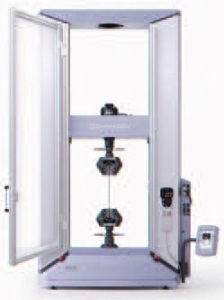Figure 1. Overview of Universal Testing System.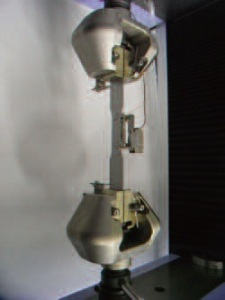Figure 2. Specimen and Jigs for tensile testing.

## Specimens and Test Conditions

Table 1 shows information on the sample specimens and Table 2 shows the test conditions.

Table 1. Sample Specimens.

Sample Name A B C D
Material Cold-rolled steel Stainless steel (austenitic) Aluminium alloy Brass
Sample size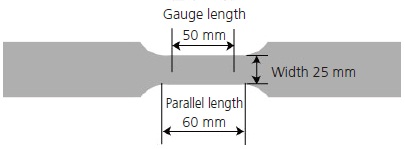Width 25 mm, thickness 1 mm, gauge length 50 mm, parallel length 60 mm (JIS Z 2241 No. 5 test specimen)

Table 2. Test Conditions.

 1) Load cell capacity 50 kN 2) Jig 50 kN Non-shift wedge type grips (file teeth for flat specimen) 3) Test speed See Table 3 4) Test temperature Ambient temperature 5) Software TRAPEZIUMX (single)

An image diagram of the test speed is shown in Figure 3 and Table 3 and Table 4 show the applicable test speeds for the strain control and stress control tests, respectively.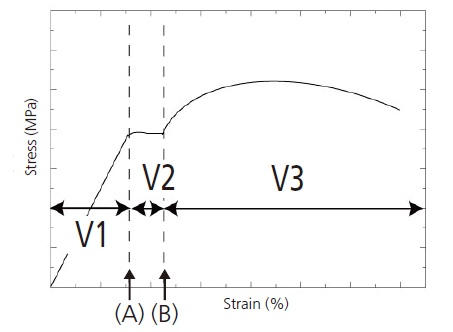(A): Upper yield point (or its corresponding point).
(B): Upper limit of strain measurement (point after proof strength point).

Figure 3. Image of Test Strain Rate.

Table 3. Test Strain Rate (based on strain rate control).

 V1: Strain rate 0.00025/s (1.5 %/min) V2: Strain rate 0.00025/s (1.5 %/min) V3: Predicted strain rate 0.0067/s (40 %/min)

Table 4. Test Stress Rate (based on stress rate control).

 V1: Stress rate 10 MPa/s V2: Strain rate 0.00083/s (5 %/min) V3: Predicted strain rate 0.0067/s (40 %/min)

Figure 4 shows the stress – stroke displacement for each sample using strain-rate control, and Table 5 shows their characteristic values. The point at which the stress becomes discontinuous, as shown by the jump in the stress-stroke displacement curve, corresponds to the point at which the strain rate is switched and this is indicated in Figure 4.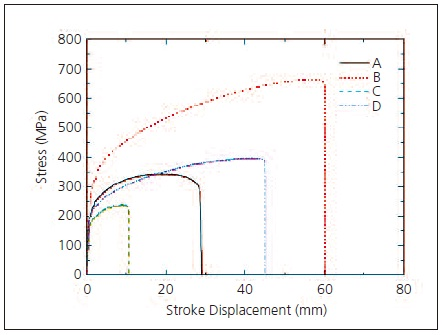Figure 4. Test Results for Each Metallic Material (stress-stroke curve based on strain rate control).

Table 5. Results (Average n=3).

Sample Name Elastic Modulus (GPa) 0.2% Proof Strength (MPa) Tensile Strength (MPa) Elongation at Break (%)
A (cold-rolled steel) 194 185.5 341.5 43.3
B (stainless steel) 200 278.5 660.8 55.0
C (aluminum) 71 170.1 236.3 13.0
D (brass) 109 193.1 398.1 49.1

Note 1) Strain rate refers to the amount of increase in strain, obtained using an extensometer to measure the gauge length of a test specimen, per unit time.
Note 2) Predicted strain rate was obtained using the displacement of the testing machine crosshead at each point in time and the test specimen's parallel length. Thus, it is defined as the increase in strain of the specimen's parallel length per unit time.

The strain rate and predicted strain rate obtained from tensile testing of the respective metallic materials using strain rate control is shown in Fig. 5 (a) – (d). The strain rate is depicted by the red solid lines, the predicted strain rate is depicted by the blue solid lines and the stress is shown by the black broken lines. Furthermore, the green dotted lines signify the permissible value ±20 % relative tolerance in the strain rate control (as specified in ISO 6892). As for the actual load rate, it is clear that the values are well within the permissible strain rate control range showing excellent strain rate control. With regards to samples A and B, displacement is measured up to 2% of the gauge length with the help of an extensometer. In case of samples C and D, the strain measured with an extensometer was only up to 0.8%, due to the formation of serration when strain corresponding to about 1% was applied.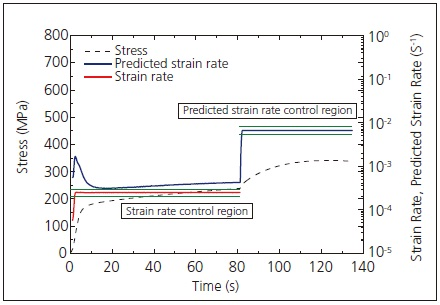Figure 5. (a) Test Results (Sample A).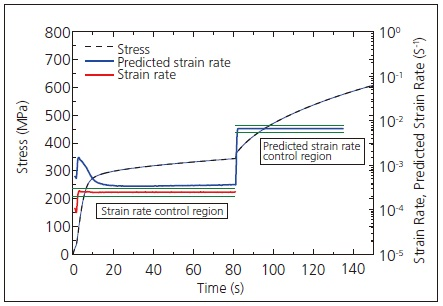Figure 5. (b) Test Results (Sample B).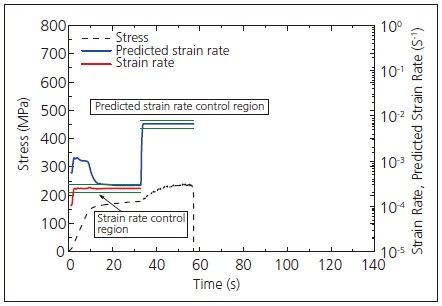Figure 5. (c) Test Results (Sample C).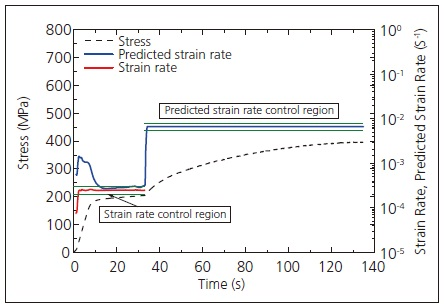Figure 5. (d) Test Results (Sample D).

The stress-stroke displacement curve diagram obtained from tensile testing of each of the metallic materials using stress rate control is shown in Figure 6 and the characteristic values are shown in Table 6.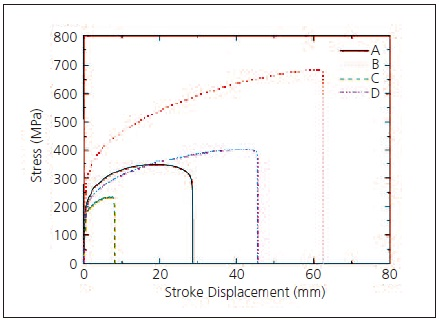Figure 6. Test Results (stress-stroke curve based on stress rate control).

Table 6. Test Results (Average n = 3).

Sample Name Elastic Modulus (GPa) 0.2% Proof Strength (MPa) Tensile Strength (MPa) Elongation at Break (%)
A (cold-rolled steel) 194 193.3 349.3 42.0
B (stainless steel) 205 290.7 687.0 54.8
C (aluminum) 69 177.0 233.5 12.6
D (brass) 112 196.7 405.5 48.8

The strain rate and predicted strain rate obtained from tensile testing of the respective metallic materials using stress rate control is shown in Figure 7. a-d. The pink solid lines show the stress rate, the blue solid lines the predicted strain rate, and the black broken lines show the stress.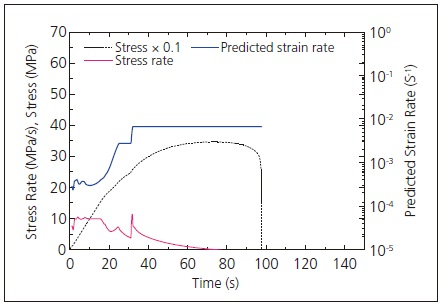Figure 7. (a) Test Results (Sample A).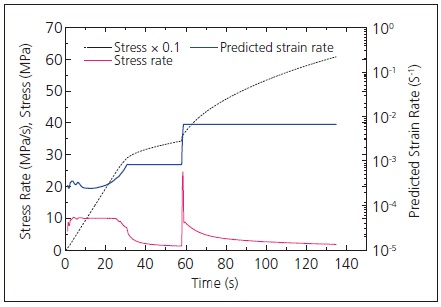Figure 7. (b) Test Results (Sample B).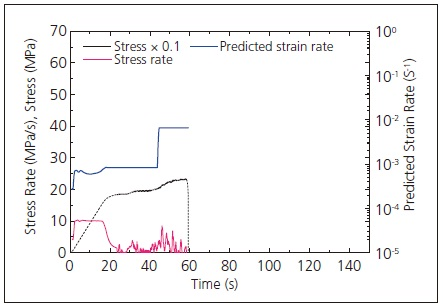Figure 7. (c) Test Results (Sample C).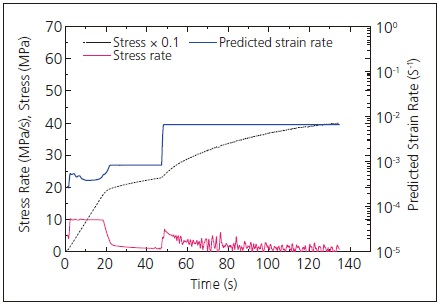Figure 7. (d) Test Results (Sample D).

## Conclusion

At the set rates, it is possible to acquire suitably stable data for predicted strain rate and stress rates. The results show that in the tensile testing of the various types of metallic materials using the Shimadzu Autograph AG-50kNX Precision Universal Tester, the strain rate control values were very much within the range of the permissible values (± 20 %) specified in ISO 6892. Likewise, stable testing using stress rate control was also achieved. While typical universal testing machines are used, testing control methods other than the crosshead rate control, e.g. strain rate control and stress rate control, normally require burdensome adjustment of the control gain based on the material being tested. However, with this instrument, strain rate control and stress rate control in tensile testing of any metallic material are easily conducted since the gain is adjusted automatically (auto-tuning feature).This information has been sourced, reviewed and adapted from materials provided by Shimadzu Scientific Instruments.

## Citations

• APA

Shimadzu Scientific Instruments. (2020, January 13). Testing the Tensile Strength of Metallic Materials. AZoM. Retrieved on October 20, 2020 from https://www.azom.com/article.aspx?ArticleID=7689.

• MLA

Shimadzu Scientific Instruments. "Testing the Tensile Strength of Metallic Materials". AZoM. 20 October 2020. <https://www.azom.com/article.aspx?ArticleID=7689>.

• Chicago

Shimadzu Scientific Instruments. "Testing the Tensile Strength of Metallic Materials". AZoM. https://www.azom.com/article.aspx?ArticleID=7689. (accessed October 20, 2020).

• Harvard

Shimadzu Scientific Instruments. 2020. Testing the Tensile Strength of Metallic Materials. AZoM, viewed 20 October 2020, https://www.azom.com/article.aspx?ArticleID=7689.

## Tell Us What You Think